# How To Use Excel Surface Chart

The surface chart is used to represent two dimensional data in 3D map. It looks like a topographical map. The higher values are shown as high peaks. It is like a curtain on a 3d column chart.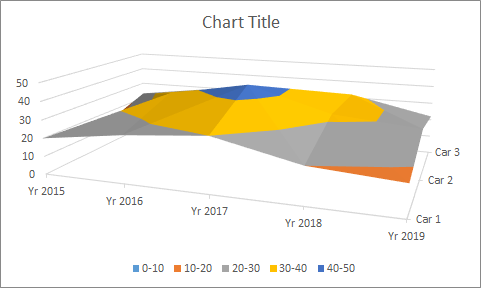The colors in the chart does not represent any legend like other chart. The colors represent the group to which that surface belongs. The Surface chart is best used when X and Y axis are numerical ranges.

There are four types of surface charts.

1. 3-D Surface chart.
2. Wireframe 3-D Surface chart.
3. Countor chart.
4. Wireframe Countor chart.

3-D Surface chart
A 3D surface chart looks like a curtain dropped on 3D  column charts. This chart is best used when x and y are numerical ranges. To understand it, let's go through an example.
Let's say we have this equation:
z= 2x3-3y2
Where -1<=x<=1 and -1<=x<=1
In above equation, x and y ranges from -1 to 1. Using this equation we have prepared a table in excel.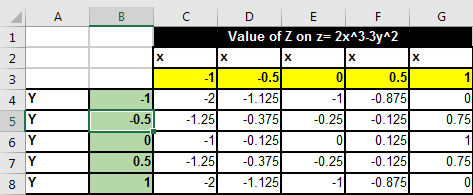To create this table we used above equation. The third row represents the values of x and B column represents values of Y, both in range -1 to 1 with an interval of .5.
To create this table, follow these steps:

• First, manually prepare the table.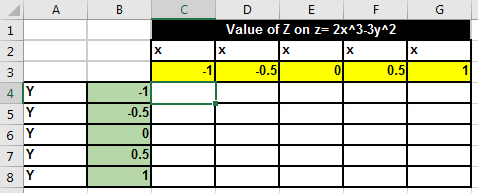• Now in cell C4 write this formula. Copy it in other cells.
=POWER(C\$3,3)-POWER(\$B4,2)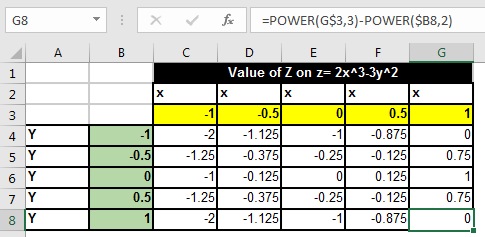Now the data is ready, let's plot an 3D area chart.

1. Select the range B3:G8.
2. Go to Insert? Charts ? Surface Charts? 3D Surface chart. And it is done.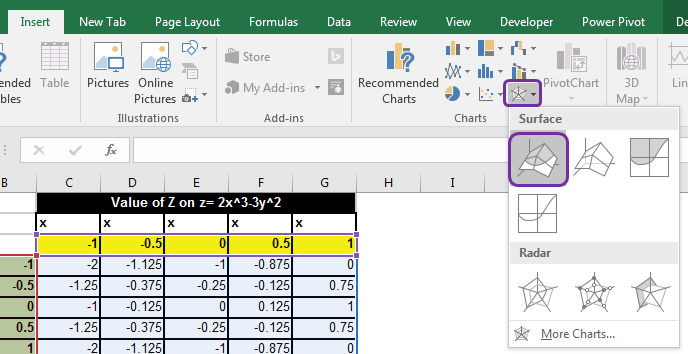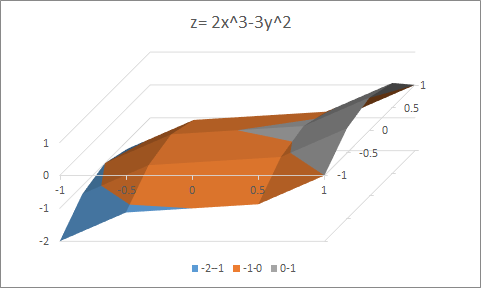Interpretation:
The colors in the chart show the data that fall between a certain range. For example, in above chart, blue color represents values between -2 to -1, orange represents -1 to 0 and grey represents 0-1.

Wireframe 3-D Surface chart
This is same as the 3-D surface chart but without filled color. It looks like frame of colored wires for 3D surface chart.
It is easy to see the backside boundaries in wireframe chart. In 3D surface chart, it was hard to see the backside area from one perspective.
To plot a wireframe surface chart, follow the exact steps as followed in 3-D surface chart. In the end choose wireframe surface chart.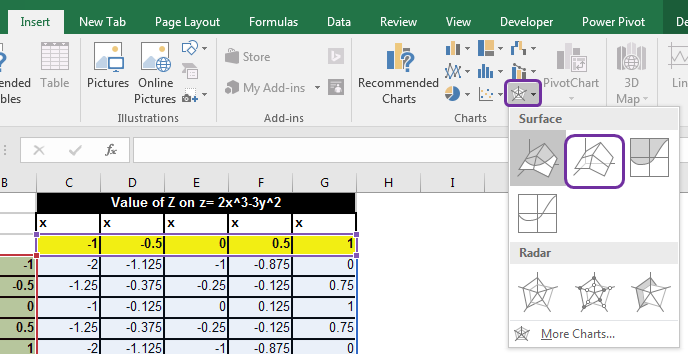You will have a chart that looks like a frame of wires.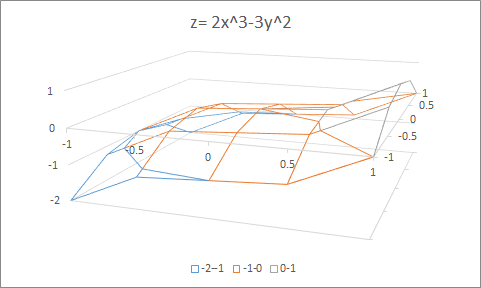Interpretation:
The interpretation is same as 3D Surface chart. In wireframe it is easy to see relations between to points on 3 dimensional surface.
Contour Chart
Contour chart is just like watching a 3D surface chart from top. In general, it is very similar to a topographical google map.
In Contour chart, the depth is not that important. You can estimate the depth by evaluating shade and light but it will be tough. The the benefit of contour chart is that you can see areas covered by other ranges.

Follow exact same steps as above and in the end choose contour chart.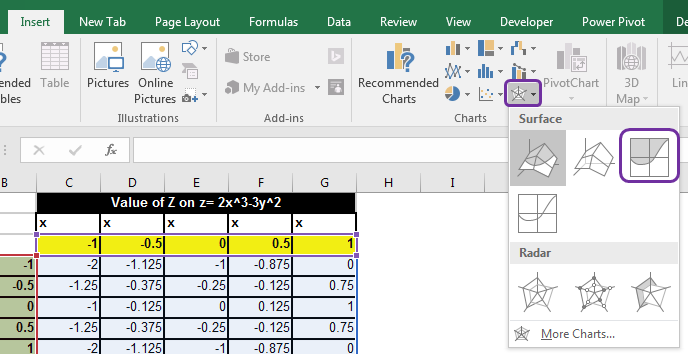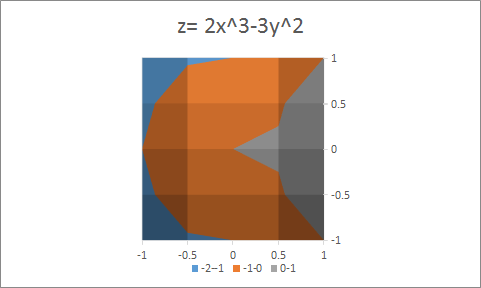Wireframe Contour Chart
The wireframe contour chart is just the contour chart without the colored areas. We can see a web of different colored wires, making a contour chart. It is like seeing a wireframe surface chart from above.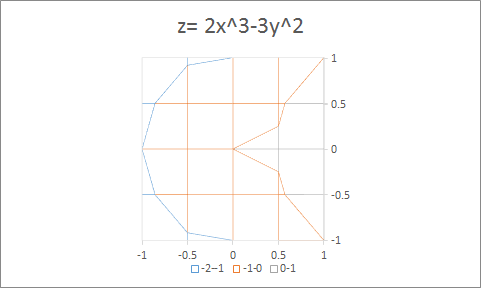So yeah guys, this how you can use different types of surface chart in excel to represent data visually. In practice, I haven't used these charts much, I may leave some points that you might know. Feel free to add those points in the comments section below. I will include that in the article with your name.

Related Articles:

Pareto Chart and Analysis

Waterfall Chart

Excel Sparklines : The Tiny Charts in Cell

Speedometer (Gauge) Chart in Excel 2016

Creative Column Chart that Includes Totals

4 Creative Target Vs Achievement Charts in Excel

Popular Articles:

The VLOOKUP Function in Excel

COUNTIF in Excel 2016

How to Use SUMIF Function in Excel

Terms and Conditions of use

The applications/code on this site are distributed as is and without warranties or liability. In no event shall the owner of the copyrights, or the authors of the applications/code be liable for any loss of profit, any problems or any damage resulting from the use or evaluation of the applications/code.